# Common Core: Kindergarten Math : The Last Number Name Said Tells the Number of Objects Counted, Regardless of Arrangement: CCSS.Math.Content.K.CC.B.4b

## Example Questions

### Example Question #21 : The Last Number Name Said Tells The Number Of Objects Counted, Regardless Of Arrangement: Ccss.Math.Content.K.Cc.B.4b

Select the answer with the same number of clouds as below.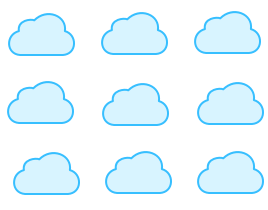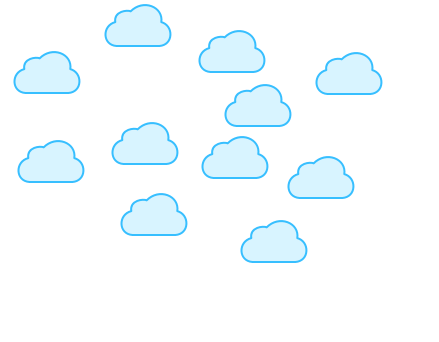Explanation:

There areclouds in the question. This picture also showsclouds.### Example Question #22 : The Last Number Name Said Tells The Number Of Objects Counted, Regardless Of Arrangement: Ccss.Math.Content.K.Cc.B.4b

Select the answer with the same number of clouds as below.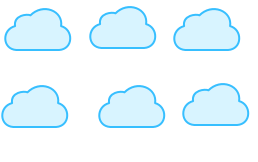Explanation:

There areclouds in the question. This picture also showsclouds.### Example Question #23 : The Last Number Name Said Tells The Number Of Objects Counted, Regardless Of Arrangement: Ccss.Math.Content.K.Cc.B.4b

Select the answer with the same number of clouds as below.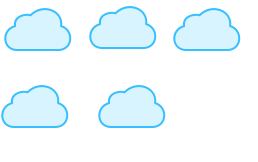Explanation:

There areclouds in the question. This picture also showsclouds.### Example Question #24 : The Last Number Name Said Tells The Number Of Objects Counted, Regardless Of Arrangement: Ccss.Math.Content.K.Cc.B.4b

Select the answer with the same number of clouds as below.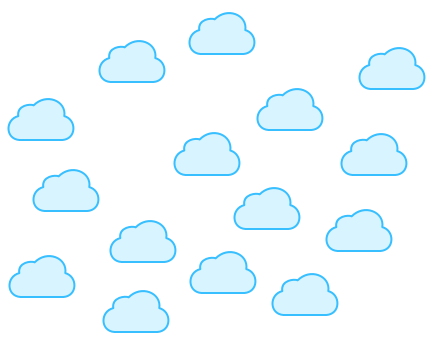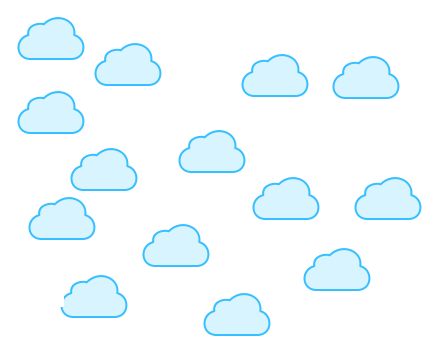Explanation:

There areclouds in the question. This picture also showsclouds.### Example Question #25 : The Last Number Name Said Tells The Number Of Objects Counted, Regardless Of Arrangement: Ccss.Math.Content.K.Cc.B.4b

Select the answer with the same number of stars as below.Explanation:

There arestars in the question. This picture also showsstars.### Example Question #26 : The Last Number Name Said Tells The Number Of Objects Counted, Regardless Of Arrangement: Ccss.Math.Content.K.Cc.B.4b

Select the answer with the same number of stars as below.Explanation:

There arestars in the question. This picture also showsstars.### Example Question #27 : The Last Number Name Said Tells The Number Of Objects Counted, Regardless Of Arrangement: Ccss.Math.Content.K.Cc.B.4b

Select the answer with the same number of stars as below.Explanation:

There arestars in the question. This picture also showsstars.### Example Question #28 : The Last Number Name Said Tells The Number Of Objects Counted, Regardless Of Arrangement: Ccss.Math.Content.K.Cc.B.4b

Select the answer with the same number of stars as below.Explanation:

There arestars in the question. This picture also showsstars.### Example Question #29 : The Last Number Name Said Tells The Number Of Objects Counted, Regardless Of Arrangement: Ccss.Math.Content.K.Cc.B.4b

Select the answer with the same number of stars as below.Explanation:

There arestars in the question. This picture also showsstars.### Example Question #30 : The Last Number Name Said Tells The Number Of Objects Counted, Regardless Of Arrangement: Ccss.Math.Content.K.Cc.B.4b

Select the answer with the same number of stars as below.There arestars in the question. This picture also showsstars.